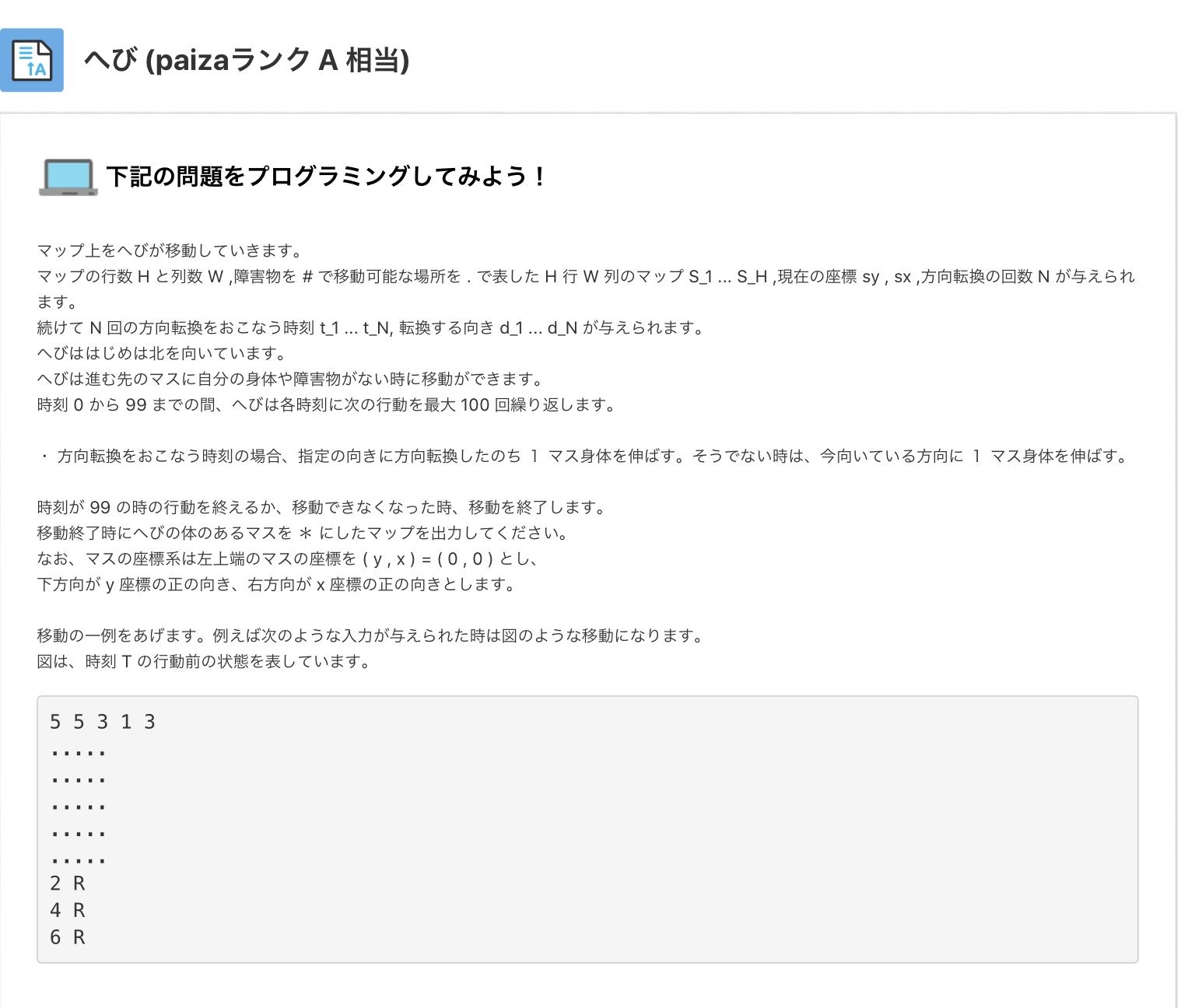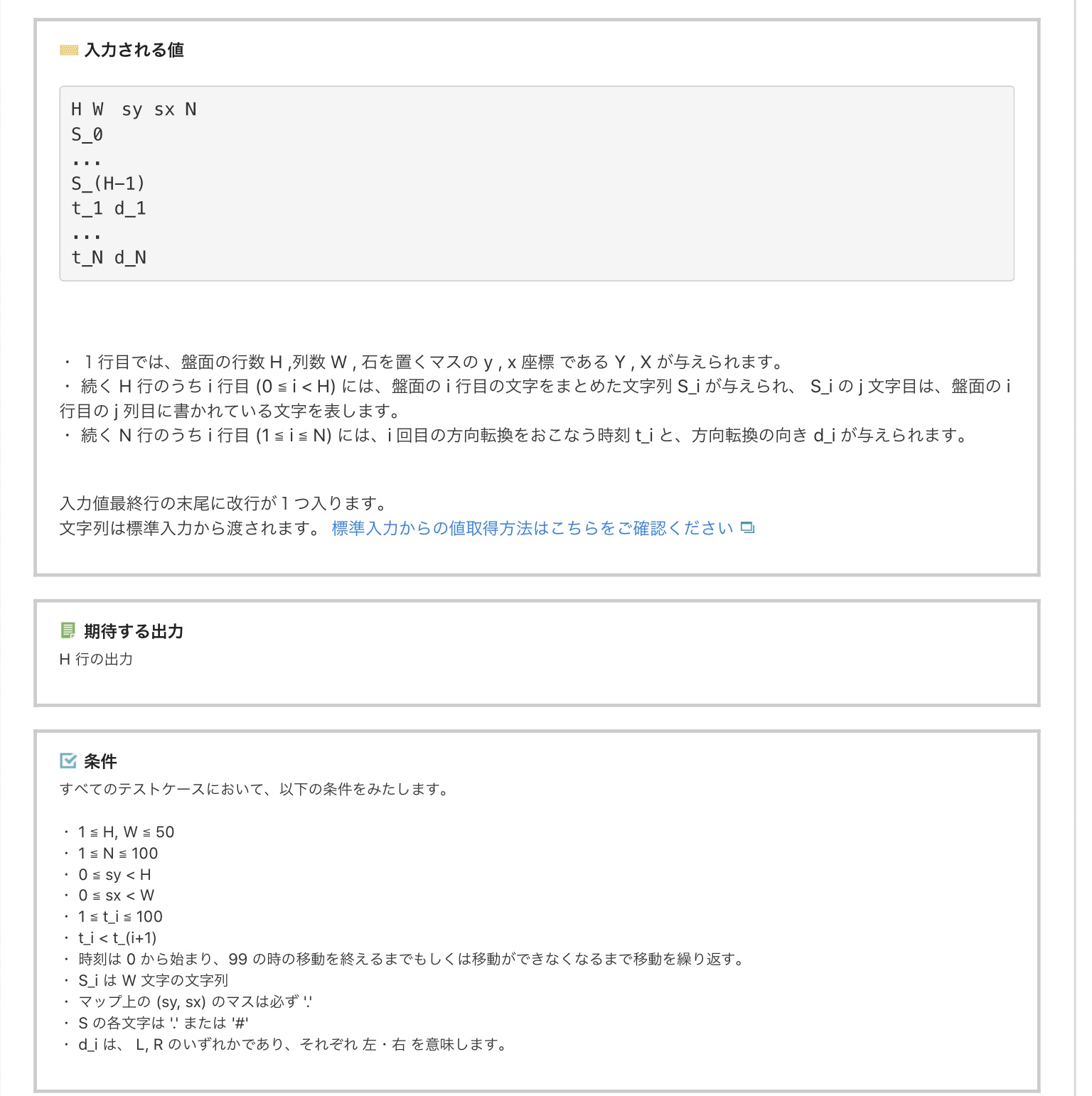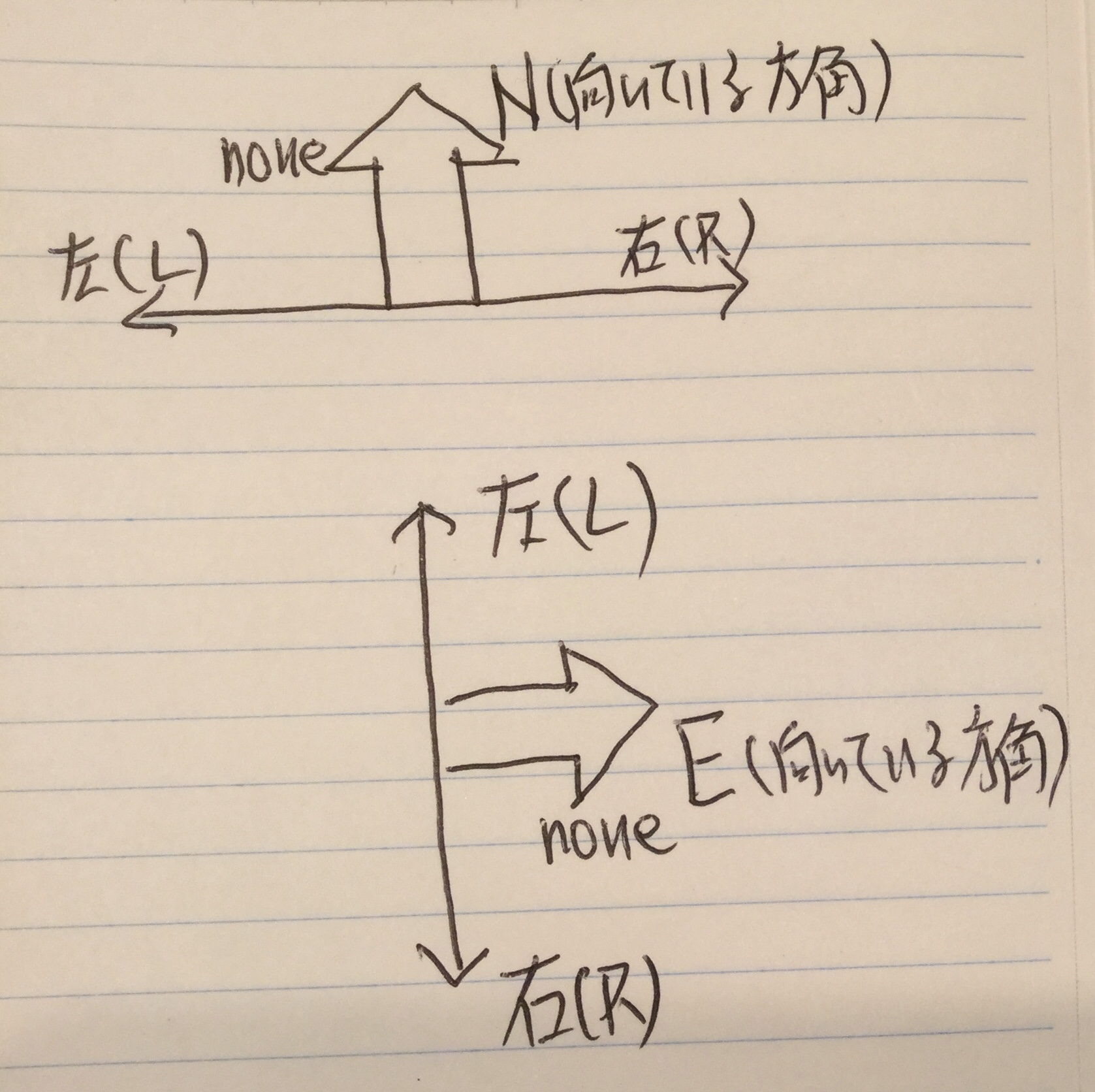# 0. Dinosaur x skill check

I haven't been able to improve my skills due to hard work these days. For some reason, I'm busier than before teleworking, even though it's been my long-cherished teleworking. And above all, the idea of a program to put out a dinosaur is disappearing. I learned more recently

## "Dinosaurs are reptiles"

Then, I found "Snake" in the skill check.

I casually liked the title I gave it and felt destined, so I wrote a process to solve it.

And the higher the rank, the longer the question sentence, and the more __ "Can you keep up with your understanding and its world view?" __ "The purpose is to solve the problem" For those who want the beauty and shortness of the code, the following sentences are unnecessary. __

Finally, I'll put a dirty code. And __ "Do not accept titles that make you feel nostalgic and cold" __ Let's start while reflecting on.

# 1. Problem "Snake"

Try pasting the problem with an image.# 2. Boundary check of destination

### 2.1 Movement range

The information displayed on the first line is the vertical and horizontal widths in which the snake can move. Let y be the vertical and x be the horizontal. 0 <= y <= H-1,0 <= x <= W-1 is the movable range.

### 2.2 Is there a wall at the destination?

The wall is represented by #, and if it is., It can be moved. What you can see here is that if 2.1 is not met, it is NG, If 2.2 is not satisfied, it is NG, and if both are satisfied, it is OK.

• As an aside, dinosaurs such as Indominas Rex break through the walls. This rule does not work. (I think I'm the rule.)

The check_limit function was created as a function to judge the above.

# 3. 3. Coordinates that transition with direction

Here, when given the direction (direction facing) and the direction to go, How much the transition destination coordinates are in the row and column directions compared to the current coordinates Move or check the next direction.

In the figure, the relationship is as follows.### 3.1 When the direction you are facing is north (N)

When moving to the right, move to the right from where you are, that is, 0 rows and 1 column. The next direction is east. When moving to the left, move to the left from where you are, that is, move 0 rows-1 columns. The next direction is west. If the orientation is not specified (this is the case when it is not the time to change direction, set to none), move up from where you are, that is, move -1 row 0 column. The next direction is north.

### 3.2 When the direction you are facing is east (E)

When moving to the right, move 1 row (bottom) 0 columns from where you are The next direction is south. When moving to the left, move -1 row (up) 0 columns from where you are. The next direction is north. When the orientation is none, move 0 rows and 1 column (right) from where you are. The next direction is east.

The check_direction function was created based on this information. (I now realize that get is better than check.)

# 4. Coordinate duplication check

The coordinates that I passed once cannot be advanced because there is already a snake's body. That thing. Apparently, it is supposed to be a huge snake like anaconda. If the transition destination has already passed, the movement will stop.

# 5. Code example

__` Overall flow` __ `(1) Get input value` `(2) Time array (tarr array) to change direction and corresponding direction array (lrarr) And the process of organizing coordinate data acquisition (arr2 array) ` `(3) Coordinate dead end judgment and creation of moving coordinates and moving direction acquisition function` `(4) Repeat the following from time 0 to 99` `(I) Find the transition destination coordinates (ny, nx) and the next direction (next_dir) for the direction facing the current coordinates (sy, sx) and the direction of travel` `(Ii) Check if the transition destination coordinates are dead ends (If there is a dead end, exit the loop)` `(Ⅲ) If it is not a dead end, check if it is the coordinates that you have passed so far` `(ⅳ) If the coordinates have not passed so far, set these coordinates as (sy, sx) and store them in snake_arr. `` `(V) In the case of the coordinates passed so far (in the case of the coordinates registered in snake_arr) Exit the loop` `(5) Assign * to the arr2 data of the coordinates registered in the snake_arr array` `(6) Output arr2`

``````
# Let's Challenge! !!

in1 = gets.chomp!
#puts(in1)
arr1=in1.split(' ')
#print(arr1)

row1=arr1.to_i
col1=arr1.to_i
sy=arr1.to_i
sx=arr1.to_i
num1=arr1.to_i

arr2 = Array.new(row1) { Array.new(col1,"") }

#print(arr2)

for i in 1..row1 do
tmp1=gets.chomp.to_s
for j in 1..col1 do
arr2[i-1][j-1]=tmp1[j-1...j]
end
end

tarr=[]
lrarr=[]

for i in 1..num1 do
tmp1=gets.chomp.to_s
arr3=tmp1.split(" ")
tarr.push(arr3.to_i)
lrarr.push(arr3)
#in3.push(tmp1)
end

def check_limit(arr0,i,j,row1,col1)
if i<0 or i>row1-1
flg=1
elsif j<0 or j>col1-1
flg=1
elsif arr0[i][j]=="#"
flg=1
else
flg=0
end
return flg
end

def check_direction(p_dir,rlstr)
retstr=""
if p_dir=="N"
if rlstr=="R"
retstr="0,1,E"
elsif rlstr=="L"
retstr="0,-1,W"
else
retstr="-1,0,N"
end
elsif p_dir=="S"
if rlstr=="R"
retstr="0,-1,W"
elsif rlstr=="L"
retstr="0,1,E"
else
retstr="1,0,S"
end
elsif p_dir=="E"
if rlstr=="R"
retstr="1,0,S"
elsif rlstr=="L"
retstr="-1,0,N"
else
retstr="0,1,E"
end
else
if rlstr=="R"
retstr="-1,0,N"
elsif rlstr=="L"
retstr="1,0,S"
else
retstr="0,-1,W"
end
end
return retstr
end

def find_lr(sarr,retarr,i2,num1)
rnum=-1
for i in 0..num1-1 do
if sarr[i]==i2
rnum=i
break
end
end
return retarr[rnum]
end

snake_arr=[]
snk_str=arr1+" "+arr1
snake_arr.push(snk_str)
#print(snake_arr)
p_dir='N'
flg_e=0
for i in 0..99 do
if tarr.count(i)==0
retstr=check_direction(p_dir,"none")
tmparr=retstr.split(",")
ny=tmparr.to_i+sy
nx=tmparr.to_i+sx
next_dir=tmparr
flg=check_limit(arr2,ny,nx,row1,col1)

else
lrstr=find_lr(tarr,lrarr,i,num1)
retstr=check_direction(p_dir,lrstr)
tmparr=retstr.split(",")
ny=tmparr.to_i+sy
nx=tmparr.to_i+sx
next_dir=tmparr
flg=check_limit(arr2,ny,nx,row1,col1)
end

if flg==0
sy=ny
sx=nx
p_dir=next_dir
str1=sy.to_s+" "+sx.to_s
if snake_arr.count(str1)==0
snake_arr.push(str1)
else
flg_e=1
#puts(1)
end
else
flg_e=1
#puts(2)
end
if flg_e==1
break
end
end

#print(snake_arr)
#print(snake_arr.length)

for i in 0..snake_arr.length-1 do
tmp1=snake_arr[i].to_s.split(" ")
arr2[tmp1.to_i][tmp1.to_i]="*"
end

str1=""

for i in 0..row1-1 do
str1=""
for j in 0..col1-1 do
str1=str1+arr2[i][j]
end
puts(str1)
end

``````